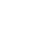I usually use the results that come from the models as objects (not as arrays). However, there are moments when I need to work with the results before sending them to the view. In this case, getting the results from the model as an array is preferable. But, when I send them to the view, I would like to work with objects. So now I need to convert the array into an object. I found the best answer to this problem on the famous stackoverflow (http://stackoverflow.com/questions/1869091/convert-array-to-object-php).

There are two solutions:

1. First one would be to iterate the array and transform it to an object:

```\$object = new stdClass();
foreach(\$array as \$key=>\$value;
{
\$object->\$key = \$value;
}```

As you can see, we made an object with stdClass, which is an empty (generic) class. And then we used the keys of the array as methods for this class, methods that will have the values that the array had.

2. The second solution, especially if you want to convert the sub-arrays recursively (in case you have a multi-dimensional array). Take note that it has a 2-3x performance hit over the looping approach (because of the sub-arrays, of course).

`\$object = json_decode(json_encode(\$array), FALSE);`

Also, I found out that you can simply convert an object into an array by type-casting it.

`\$array = (array) \$object;`

Please, refrain from comments like: “Don’t you know you can also retrieve results as array from the model?”. Yes. I know I can.

## One comment

1.FERMAX says:

Thank you for this useful information !!!!!